# Inter maths solutions for IIA Random Variables and Probability Distributions

Intermediate mathematics IIA chapter 10 Random Variables and Probability Distrbutions exercises 10(a) and 10(b) solutions are given.

These solutions are very easy to understand. First study the text book lessons very well. Then observe solutions and try them in your own method.

You can also see the solutions for  inter first year, some chapters for Inter second year, 10th class maths and ncert maths.

You can also see

Inter Maths 1A textbook solutions

Inter Maths 1B textbook solutions

Inter Maths IIA textbook solutions

Inter Maths IIB textbook solutions

M

# Random Variables and Probability Distributions solutions Inter

Random Variables and Probability Distributions

CHAPTER – 10,Exercise 10(a)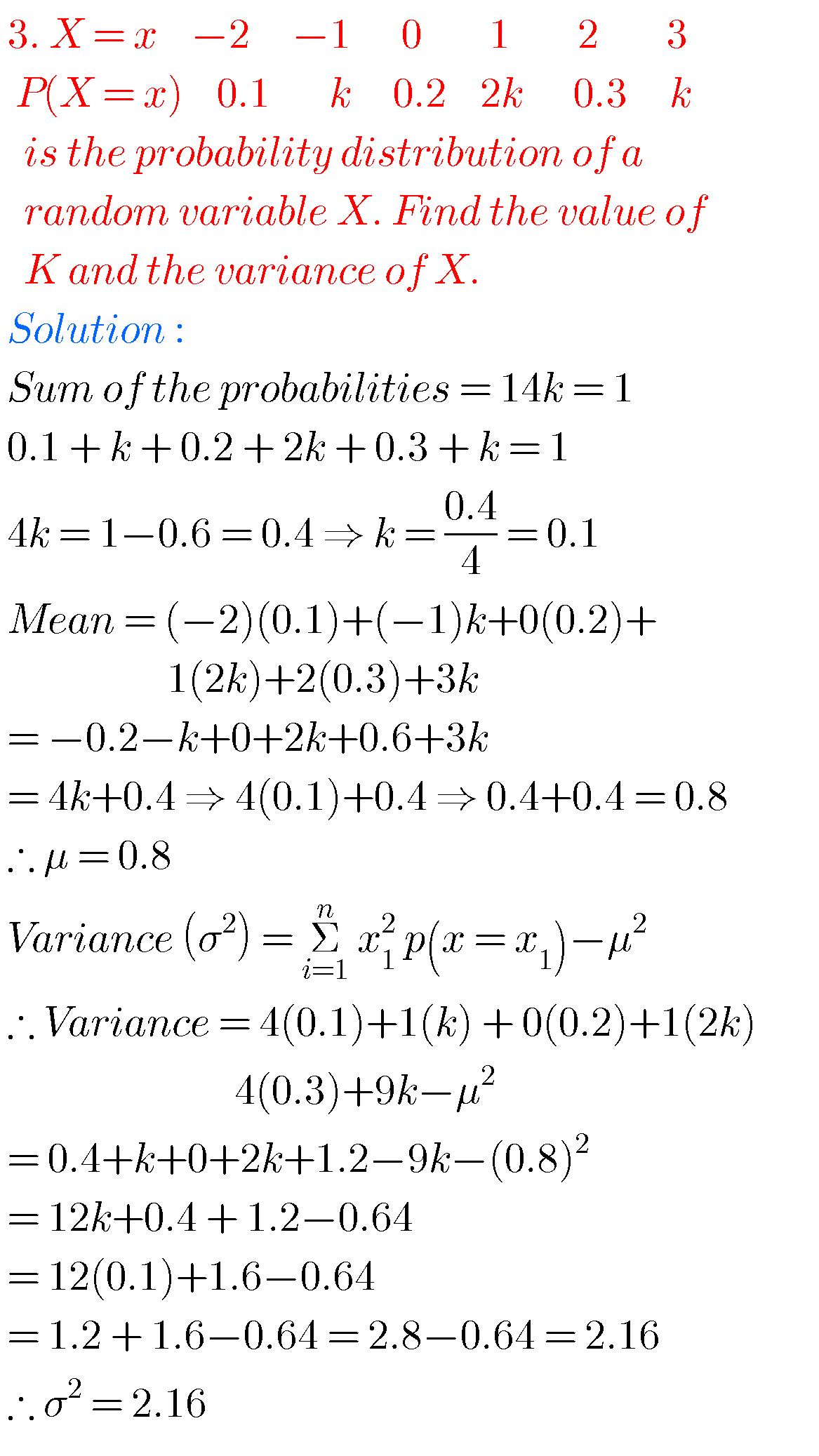##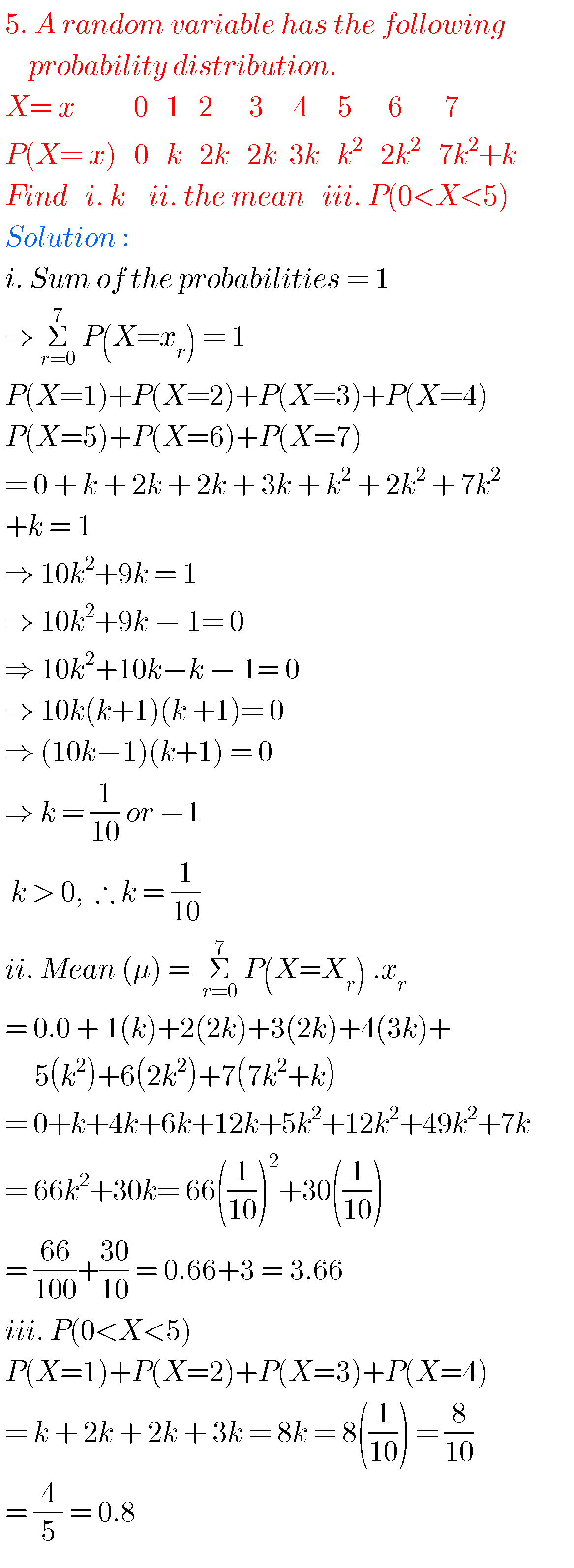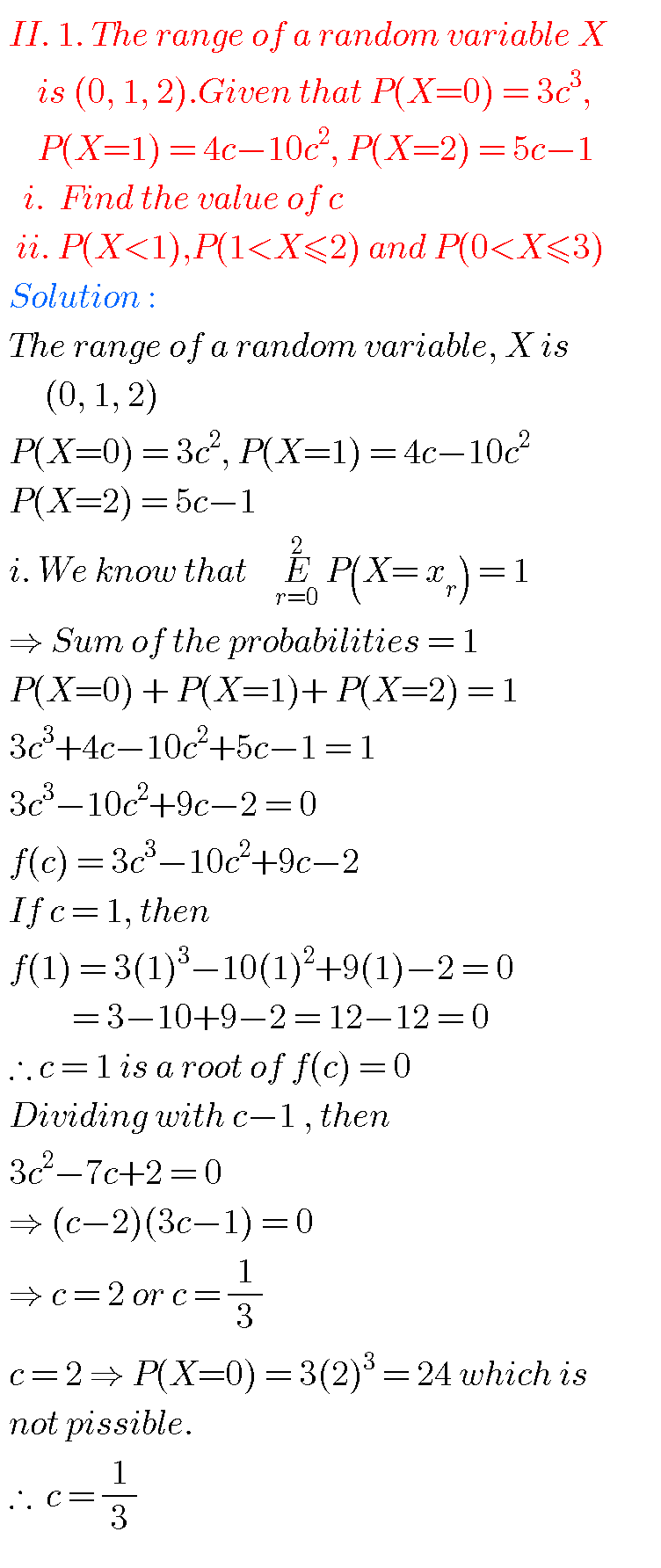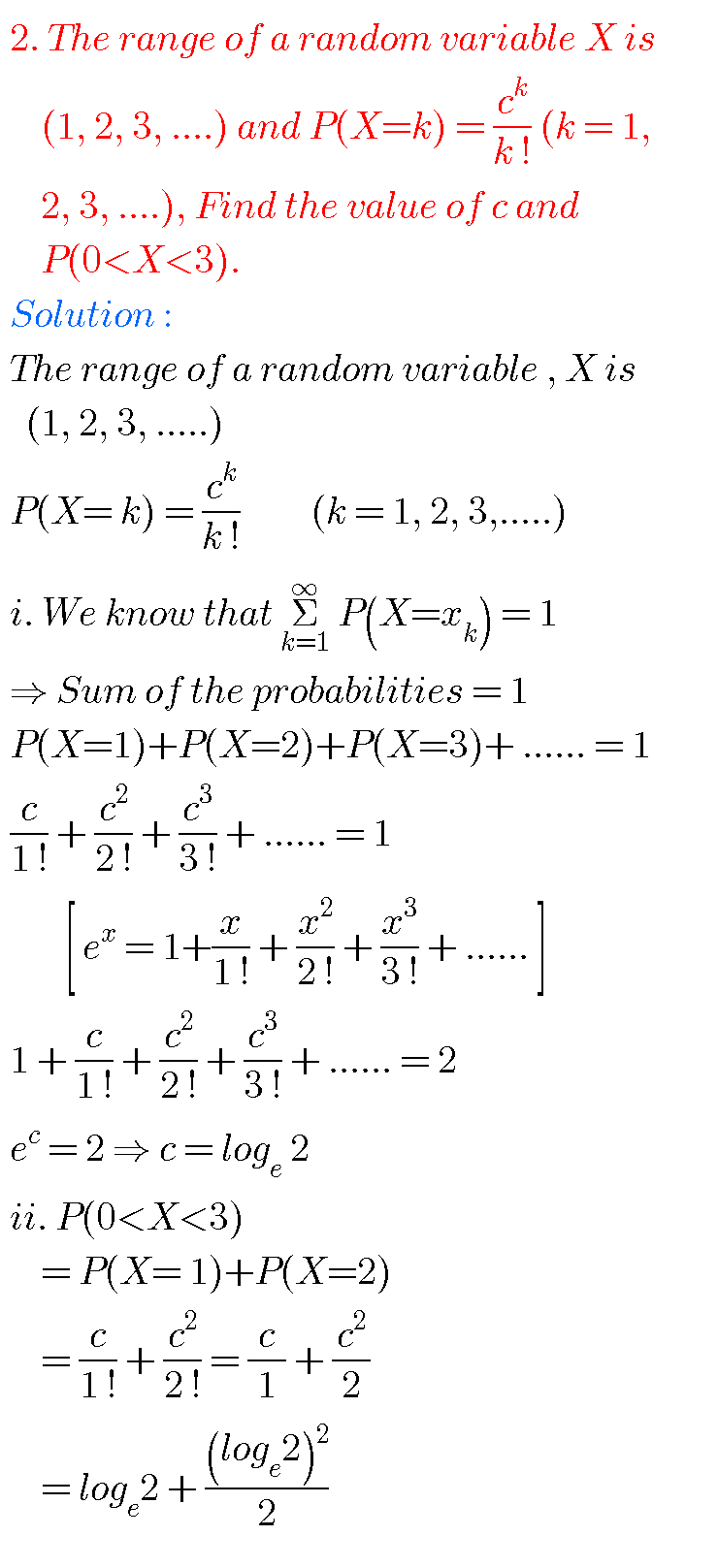## Maths inter solutions for random variables and distributions solutions

CHAPTER – 10, Exercise 10(b)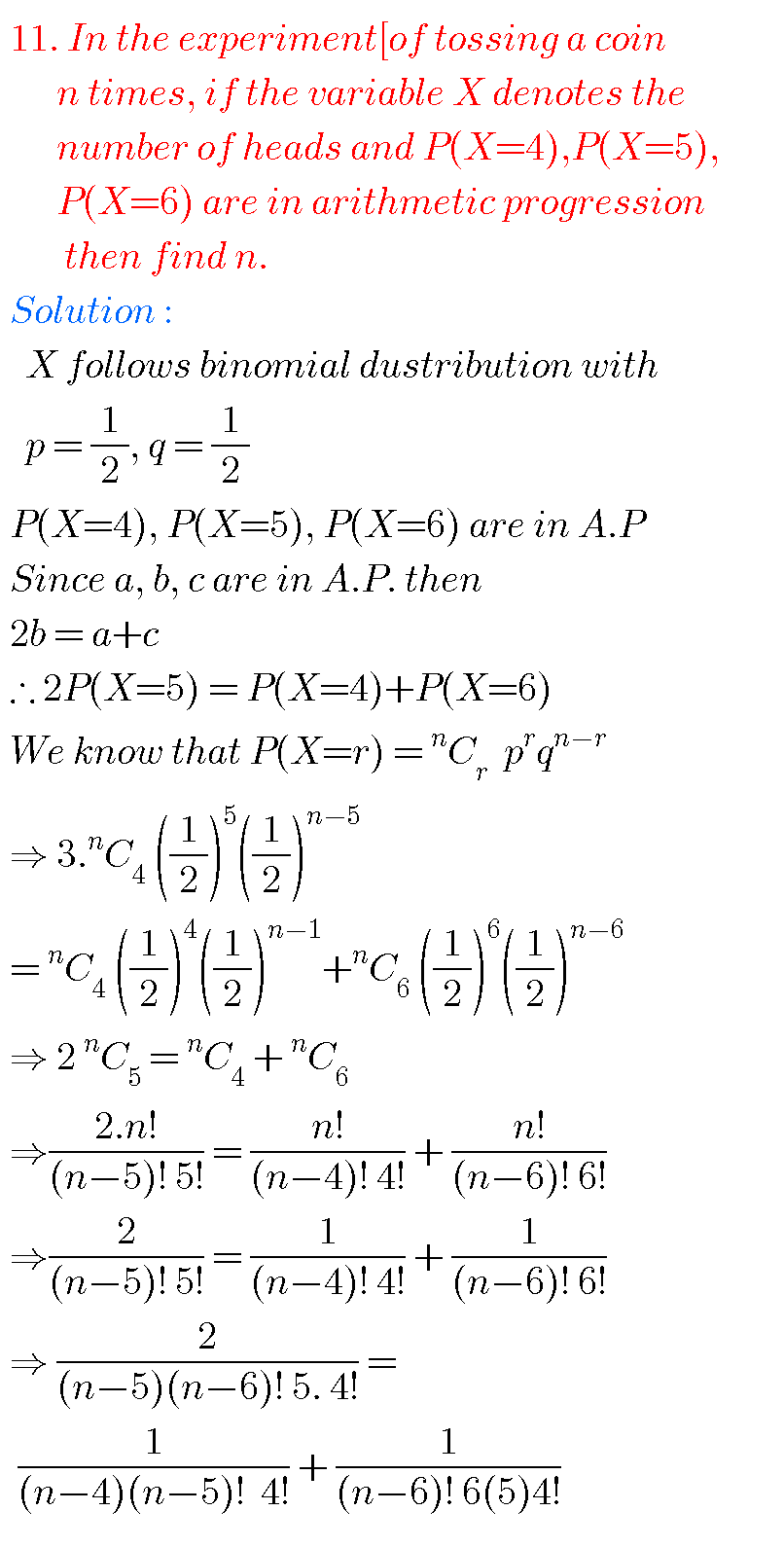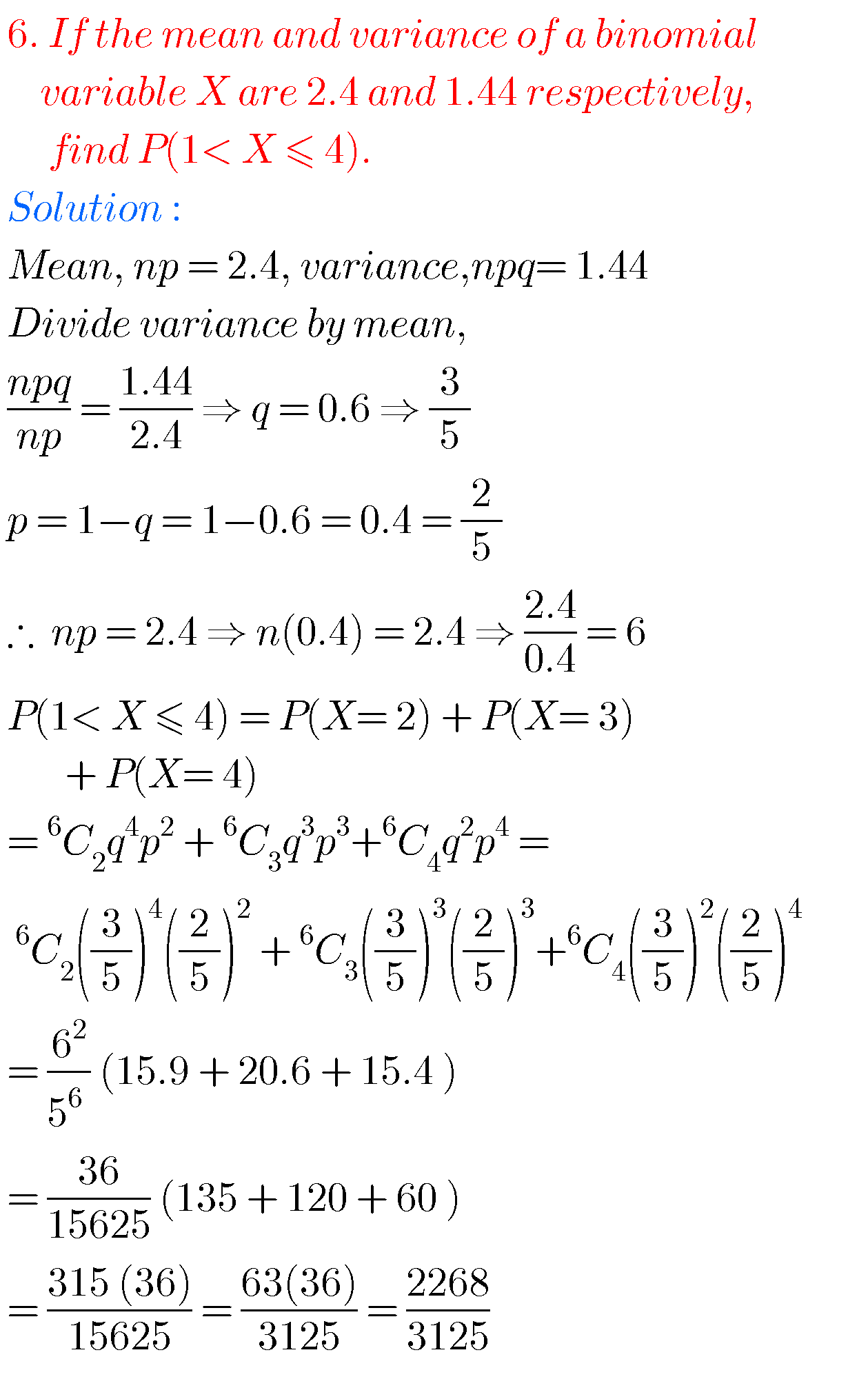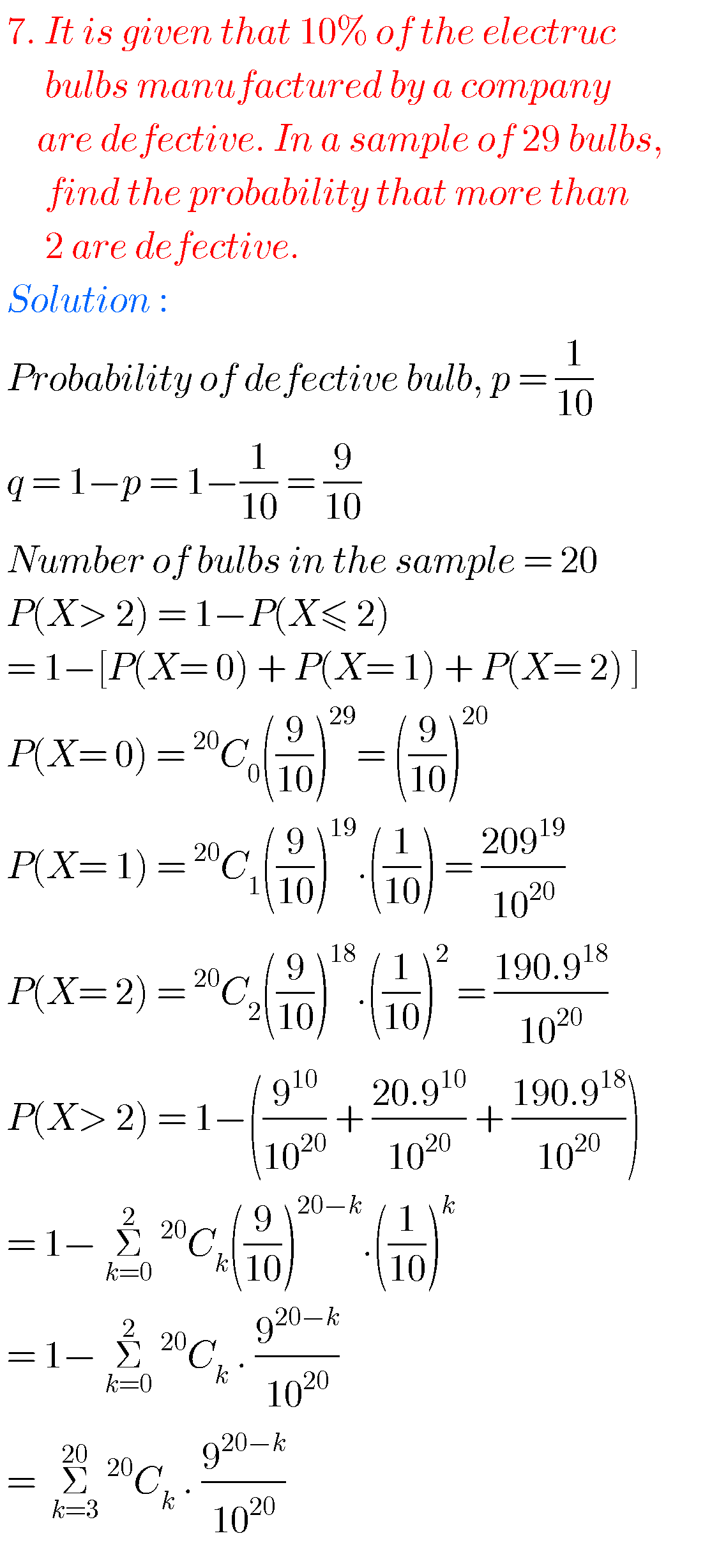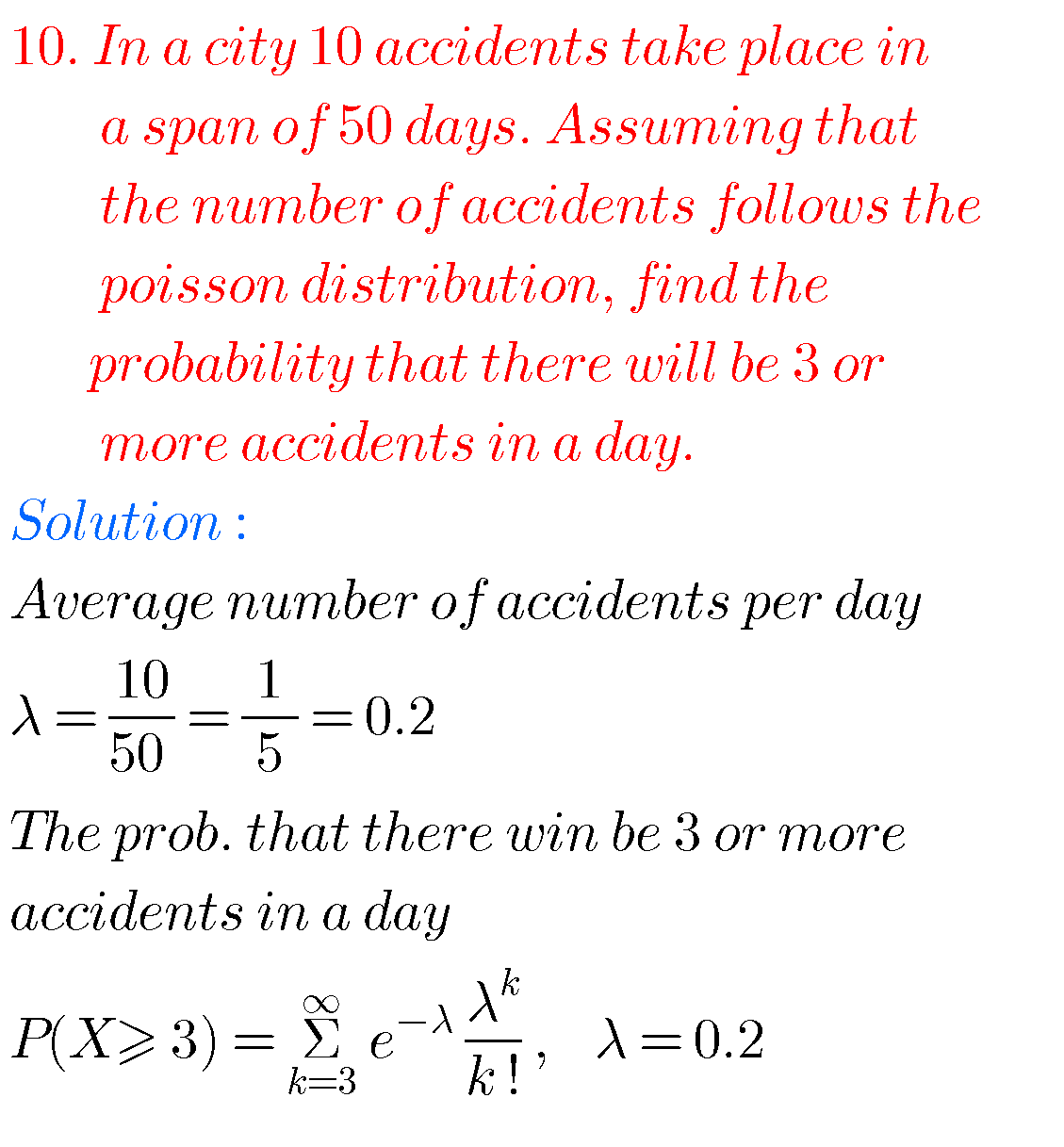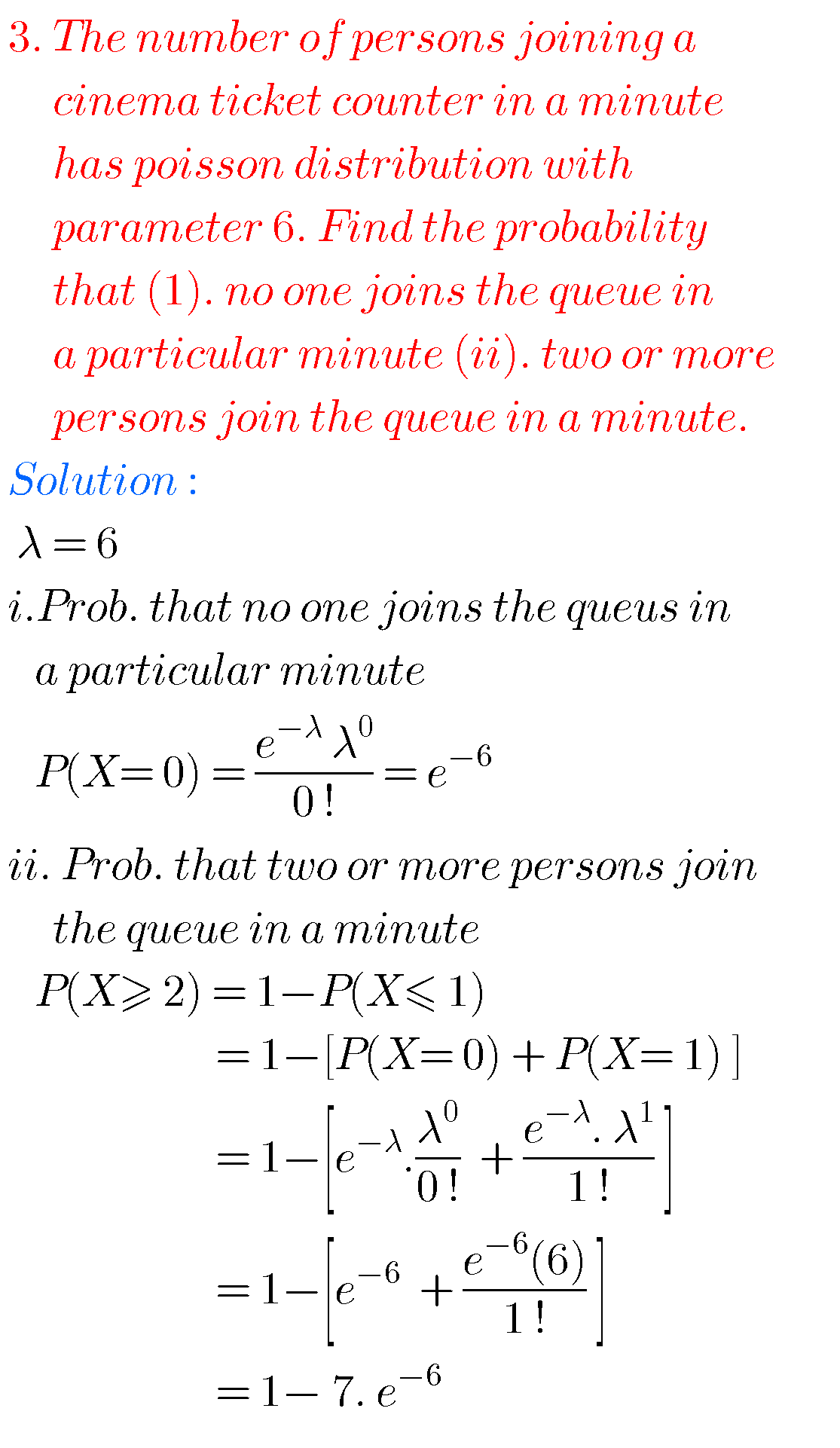Note : Observe the solutions and try them in your own methods.

class 10 maths polynomials solutions

Ncert maths class 8 exponents and powers

Ncert maths class 7 fractions and decimals

Ncert maths class 6 playing with numbers